• ## 表示学习

千次阅读 2018-05-15 17:40:44
表示学习——回顾与展望摘要——机器学习算法的成功通常取决于数据表示，并且我们假设这是因为不同的表示可能会或多或少地隐藏和隐藏数据背后变化的不同解释因素。尽管可以使用特定的领域知识来帮助设计表示，但也...
表示学习——回顾与展望摘要——机器学习算法的成功通常取决于数据表示，并且我们假设这是因为不同的表示可能会或多或少地隐藏和隐藏数据背后变化的不同解释因素。尽管可以使用特定的领域知识来帮助设计表示，但也可以使用通用的先验知识进行学习，而对AI的追求则激励设计更强大的表示学习算法来实现这样的先验知识。 本文回顾了近期在无监督特征学习和深度学习方面的工作，包括概率模型，自动编码器，流形学习和深度网络。这激发了关于学习良好表示，计算表示（即推论）以及表示学习，密度估计和流形学习之间的几何关系的长期未解答的问题。 关键词——深度学习，表示学习，无监督学习，玻尔兹曼机，自编码，神经网络 1. 导论机器学习方法的性能在很大程度上取决于它们所应用的数据表示（或特征）的选择。出于这个原因，部署机器学习算法的实际工作大部分都集中在预处理流水线和数据转换的设计上，导致数据表示可以支持有效的机器学习。这样的特征工程很重要，但是需要大量劳动力，突出了当前学习算法的弱点：他们无法从数据中提取和组织信息。特征工程是一种利用人类独创性和先前知识来弥补这一弱点的方法。为扩大机器学习的适用范围和易用性，非常希望使学习算法更少地依赖于特征工程，从而可以更快地构建新的应用程序，更重要的是，可以朝向人工智能（AI）迈进。人工智能必须从根本上理解我们周围的世界，并且我们认为只有当它能够学会识别和分解隐藏在观察到的低级别感官数据环境中的潜在解释性因素时才能实现。本文关于表示学习，即学习数据的表示，以便在构建分类器或其他预测器时更容易提取有用的信息。在概率模型的情况下，好的表示通常是捕获观察输入的基本解释性因素的后验分布。一个好的表示也是一个有用的监督预测器的输入。在各种学习表征方式中，本文重点介绍深度学习方法：通过多个非线性变换的组合形成的方法，其目标是产生更抽象的，最终更有用的表示。在这里，我们调查这个快速发展的地区，特别强调最近的进展。我们考虑一些推动该领域研究的基本问题。具体来说，是什么让一个表示比另一个更好？举一个例子，我们应该如何计算它的表示，即执行特征提取？另外，学习好的表示有什么合适的目标？ 2. 为什么我们应该关注表示学习？2.1   语音识别和信号处理2.2   目标识别2.3   自然语言处理（NLP）2.4   多任务迁移学习，领域适应 3. 怎样才能有好的表示3.1   AI中表示学习的先验知识3.2   平滑度与维数灾难3.3   分布式表示3.4   深度与抽象3.5   解决变异因子（Disentangling Factors of Variation）3.6   表示学习的好的标准4. 建立深度表示5. 单层次学习模型5.1 主成分分析（PCA）6. 概率模型6.1   有向图模型6.1.1 概率挤压（Explaining Away）6.2   无向图模型6.3   受限玻尔兹曼机（RBM）到真值数据的泛化6.4   RBM参数评估6.4.1  CD评估6.4.2  SML算法6.4.3  伪相似性，比率匹配等7. 直接学习从输入到表示的映射参数7.1   自编码7.2   规范化自编码7.2.1  稀疏自编码7.2.2  DAEs7.2.3  CAEs7.2.4  PSD8. 流形学习的表示学习8.1   基于邻居图学习一个参数映射8.2   学习表示非线性流形8.3   扩充模式化正切空间9. 概率模型和直接编码模型的联系9.1   PSD：概率解释9.2   用规范化自编码捕获维度的局部结构9.3   学习近似推理9.4   抽样挑战9.5   评估与监测性能10.深度模型的全局训练10.1  训练深度构架的挑战10.2  DBMs的联合训练10.2.1 平均场的近似推理10.2.2 训练DBMs11.基于不变性11.1  生成变形的实例11.2  卷积和池化11.2.1 基于块的训练11.2.2 卷积和平铺卷积训练11.2.3 转换成池化11.3  时序耦合和慢特征11.4  解决变异因子的算法12.结论   参考文献Representation Learning:A Review and New PerspectivesYoshuaBengio, Aaron Courville, and Pascal Vincent.IEEE TPAMI VOL. 35, NO. 8,AUGUST 2013
展开全文深度学习 无监督学习 神经网络
• ## 知识表示学习模型

万次阅读 2019-10-01 13:43:34
写在前面 最近清华的THUNLP整理了Pre...本来这篇是打算写一写Knowledge Graph + BERT系列工作的，但是最近有在做知识图谱的一些东西所以就先整理一下知识表示学习(knowledge representation learning)的相关模型发展...
欢迎关注我的微信公众号：NewBeeNLP，及时获取更多干货资源。
最近清华的THUNLP整理了Pre-trained Languge Model (PLM)相关的工作：PLMpapers，非常全面，想要了解最新NLP发展的同学不要错过。本来这篇是打算写一写Knowledge Graph + BERT系列工作的，但是最近有在做知识图谱的一些东西所以就先整理一下**知识表示学习(knowledge representation learning)**的相关模型发展，这样我们看后面的paper也会得心应手一些。1. A glance at Knowledge Representation Learning
What
首先什么是表示学习？其实我们早就在频繁使用了，比如词向量embedding，这种将研究对象的语义信息表示为稠密低维的实值向量的形式就叫表示学习。
有了表示学习的定义，那么知识表示学习(KRL) 就是面向知识库中实体和关系的表示学习，通过将实体或关系投影到低维向量空间，能够实现对实体和关系的语义信息的表示，可以高效地计算实体、关系及其之间的复杂语义关联。
Why
那么为什么会冒出知识表示学习呢？

显著提高计算效率。 简单地基于图算法计算实体间的语义和推理关系，其计算复杂度高、可扩展性差；而表示学习得到的分布式表示，则能够高效地实现语义相似度计算等操作。
有效缓解数据稀疏。 一方面将每个对象投影到低维的稠密向量空间；另一方面在投影过程中也可以借用高频对象的语义信息帮助低频对象的语义表示，捕获长尾分布。
实现异质信息融合。 将不同来源的对象投影到同一语义空间中，就能够建立统一的表示空间。

How
接下去会介绍知识表示学习的主要方法及前沿进展，这也是本系列博文的重要。首先给出整体定义：

知识库表示为 G=(E, R, S)，其中$E={\{e_{1},e_{2}, \cdots, e_{E}\}}$是实体集合，$R=\left\{r_{1}, r_{2}, \cdots, r_{R}\right\}$是关系集合，S是三元组集合，表示为(h, r, t)

基于距离的模型
基于翻译的模型
语义匹配模型
融合多源信息的模型
前沿进展

paper list ref

东南大学《知识图谱》课程

2. Distance-Based Models
UM Joint Learning of Words and Meaning Representations for Open-Text Semantic Parsing(2012)SELearning Structured Embeddings of Knowledge Bases(AAAI/2011)
在本文中，作者指出目前已有的知识库都是基于不同的框架，因此很难将它们整合应用到一个新的系统中。但是知识库中存在大量的结构化和组织化的数据，如果能够充分利用起来将会对AI领域非常有帮助。于是作者们提出一种模型将任何知识库中的实体和关系嵌入到一个更灵活的连续向量空间。嵌入可以认为是一个神经网络，该神经网络的特殊结构允许将原始数据结构集成到所学习的表示中。更准确地说，考虑到知识库是由一组实体和它们之间的关系定义的，该模型为每个实体（即一个低维向量）学习一个嵌入(embedding)，为每个关系（即矩阵）学习一个运算符(operator)。另外，在低维embedding空间使用核密度估计可以估量空间的概率密度，这样可以量化实体之间的关系存在可能性。
结构表示（Structured Embeddings，SE）中每个实体用$d$维的向量表示，所有实体被投影到同一个$d$维向量空间中。同时，SE还为每个关系定义了两个矩阵$\boldsymbol{M}_{r, 1}, \boldsymbol{M}_{r, 2} \in \mathbb{R}^{d \times d}$用于三元组中头实体和尾实体的投影操作。最后SE为每个三元组$(h, r, t)$定义了损失函数：
$f_{r}(h, t)=\left|\boldsymbol{M}_{r, 1} \boldsymbol{l}_{h}-\boldsymbol{M}_{r, 2} \boldsymbol{l}_{t}\right|_{L_{1}}$对于上述损失函数，我们可以理解为通过两个关系矩阵将头尾两个实体投影到同一关系空间中，然后在该空间中计算两投影向量的距离。而这个距离则反映了头尾实体之间在特定关系下的语义相似度，他们的距离越小则表明越有可能存在这种关系。
SE模型要学习的参数是实体向量矩阵$E$ 和两个关系矩阵$R^{lhs}$、$R^{rhs}$，下面是模型的训练过程：可以看出SE模型对头尾两个实体使用不同的矩阵进行投影，协同性较差，往往无法精确刻画两实体之间的语义联系。

3.  Trans-Based Models
TransE Translating Embeddings for Modeling Multi-relational Data(NIPS2013)
TransE是Trans系列模型的开山之作，也是知识图谱向量表示的baseline模型，后面会介绍它的很多变体。其实TransE的思想很简单，为了将实体与关系嵌入到低维的向量空间，对于知识库中的每个三元组$(h, r, t)$，认为头实体的embedding加上关系的embedding之后应该是与尾实体的embedding非常接近的。于是，可以将关系看做是两个实体之间的某种平移向量，如下图：按照上面的思路，即有了TransE模型的损失函数：$\mathcal{L}=\sum_{(h, \ell, t) \in S\left(h^{\prime}, \ell, t^{\prime}\right) \in S_{(h, \ell, t)}^{\prime}}\left[\gamma+d(\boldsymbol{h}+\ell, \boldsymbol{t})-d\left(\boldsymbol{h}^{\prime}+\ell, \boldsymbol{t}^{\prime}\right)\right]_{+}$这个损失函数是带有negative sampling和margin-based loss的

negative sampling：$S_{(h, \ell, t)}^{\prime}=\left\{\left(h^{\prime}, \ell, t\right) | h^{\prime} \in E\right\} \cup\left\{\left(h, \ell, t^{\prime}\right) | t^{\prime} \in E\right\}$表示负样本三元组，构造的方法是将S中每个三元组的头实体、关系和尾实体其中之一随机替换成其他的实体或关系，注意每个三元组只能同时替换一个元素。
margin-based loss：为了增强知识表示的区分能力，采用了最大间隔的方法，可以类比SVR的损失函数。$d(\boldsymbol{h}+\ell, \boldsymbol{t})$表示正样本的不相似得分，$d\left(\boldsymbol{h}^{\prime}+\ell, \boldsymbol{t}^{\prime}\right)$表示负样本的不相识得分，最小化这个损失函数可以使得正样本的得分越来越高而负样本的得分越来越低，并且只有两个得分差距大于某一阈值的时候loss才为0。

最终模型整体的训练过程如下：虽然TransE简单有效，但仍然存在一个非常严重的问题：不能很好处理复杂关系。举个栗子，有两个三元组(美国，总统，奥巴马)和(美国， 总统， 布什)，使用TransE模型表示的话会得到奥巴马和布什的表示向量非常接近，甚至完全相同。Code Here

TransHKnowledge Graph Embedding by Translating on Hyperplanes(AAAI2014)
相对于之前的知识表示模型，TransE有了很大的改进，但是当面对自反关系、一对多、多对一以及多对多这类复杂的关系时，就很难处理好（会使得一些不同的实体具有相同或者相近的向量表示）。于是作者提出TransH模型，在保证模型complexity和efficiency的同时，解决上述复杂关系的表示。
模型
TransH模型的主要思想是对每一个关系定义两个向量：超平面$w_{r}$和关系向量$d_{r}$。对于任意一个三元组$(h, r, t)$，$h$和$t$在超平面上的投影分别为$h_{\perp}$和$t_{\perp}$，如果是golden triplet，则存在$h_{\perp}+d_{r} \approx t_{\perp}$。看下图可能会更清楚一些：损失函数与TransE的一样：$\mathcal{L}=\sum_{(h, r, t) \in \Delta\left(h^{\prime}, r^{\prime}, t^{\prime}\right) \in \Delta_{(h, r, t)}^{\prime}}\left[f_{r}(\mathbf{h}, \mathbf{t})+\gamma-f_{r^{\prime}}\left(\mathbf{h}^{\prime}, \mathbf{t}^{\prime}\right)\right]_{+}$$f_{r}(\mathbf{h}, \mathbf{t})=\left\|\left(\mathbf{h}-\mathbf{w}_{r}^{\top} \mathbf{h} \mathbf{w}_{r}\right)+\mathbf{d}_{r}-\left(\mathbf{t}-\mathbf{w}_{r}^{\top} \mathbf{t} \mathbf{w}_{r}\right)\right\|_{2}^{2}$$\mathbf{h}_{\perp}=\mathbf{h}-\mathbf{w}_{r}^{\top} \mathbf{h} \mathbf{w}_{r}, \quad \mathbf{t}_{\perp}=\mathbf{t}-\mathbf{w}_{r}^{\top} \mathbf{t} \mathbf{w}_{r}$
模型整体训练过程也同TransE一样，这里就不再赘述。
负采样策略
不同于TransE模型中的随机替换头实体和尾实体之一作为负样本的方法，在TransH中指出：

However, as a real knowledge graph is often far from completed, this way of randomly sampling may introduce many false negative labels into training.

于是，在替换头实体或者尾实体的时候考虑了一个采样概率，$\frac{t p h}{t p h+h p t}$的概率替换头实体，$\frac{h p t}{t p h+h p t}$的概率替换尾实体，其中$tph$为每个头实体对应尾实体的平均数量，$hpt$为每个尾实体对应头实体的平均数量。也就是说，对于一对多的关系，更大的概率替换头实体；对于多对一的关系，更大概率替换尾实体。
Code Here

TransR Learning Entity and Relation Embeddings for Knowledge Graph Completion(AAAI2015)
前面介绍的TransE和TransH都是假设三元组的实体和关系在同一语义空间中的，尽管在TransH中采用了关系超平面的策略，但由于实体和关系是完全不同的，每一个实体可能会有多个aspect，即是是同一个实体在不同的关系下关注的可能是完全不相关的aspect，因此之前的模型还是不足以准确地表示knowledge graph。
于是，在本文中作者提出一种新模型TransR，其思想就是将实体和关系的表示空间区分开来，实体有entity space，关系有relation space，在计算时通过一个投射矩阵$M_{r}$将实体映射到关系空间进行计算。
$投影过程：\mathbf{h}_{r}=\mathbf{h} \mathbf{M}_{r}, \quad \mathbf{t}_{r}=\mathbf{t} \mathbf{M}_{r}$$打分函数： f_{r}(h, t)=\left\|\mathbf{h}_{r}+\mathbf{r}-\mathbf{t}_{r}\right\|_{2}^{2}$$损失函数：L=\sum_{(h, r, t) \in S\left(h^{\prime}, r, t^{\prime}\right) \in S^{\prime}} \max \left(0, f_{r}(h, t)+\gamma-f_{r}\left(h^{\prime}, t^{\prime}\right)\right)$此外，不仅仅实体有多个aspect，对于关系，也可能存在多义性，比如关系（location location contains）其实包含country-city、country-university、continent-country等多种含义。为此，作者们提出CTransR，即Cluster-based TransR，对同一关系下的不同实体对进行聚类并学习到关系在不同聚类簇的表示。具体做法是

利用TransE训练$(h, r, t)$三元组
利用vector offset $(h-t)$进行聚类
对每一类的子关系都学习到一个变换矩阵$M_{r}$和表示向量$r_{c}$
$f_{r}(h, t)=\left\|\mathbf{h}_{r, c}+\mathbf{r}_{c}-\mathbf{t}_{r, c}\right\|_{2}^{2}+\alpha\left\|\mathbf{r}_{c}-\mathbf{r}\right\|_{2}^{2}$

Code Here

TransDKnowledge graph embedding via dynamic mapping matrix(ACL2015)
文章首先指出了TransR的三个问题：

在TransR中，对于特定关系，所有实体共享同一映射矩阵。但是实际情况头尾实体可能属于不同范畴，例如(美国，总统，特朗普)这个三元组中，美国和特朗普就不是相同范畴的实体，因此应该采用不同的映射矩阵；
投影运算是实体与关系的交互过程，映射矩阵仅由关系确定是不合理的；
矩阵向量乘法运算量大，当关系数大时，矩阵向量乘法的参数也比TransE和TransH多。

基于此，作者们提出一种基于动态映射矩阵的TransD模型。对于每个三元组$(h, r, t)$，其中每个元素都有两种向量表示，一种是构建动态映射矩阵向量$(h_{p}, r_{p}, t_{p})$；另外一种是用于自身实体/关系语义表示$(\bold h, \bold r, \bold t)$。首先是利用头尾实体的映射向量构建映射矩阵：\begin{aligned} \mathbf{M}_{r h} &=\mathbf{r}_{p} \mathbf{h}_{p}^{\top}+\mathbf{I}^{m \times n} \\ \mathbf{M}_{r t} &=\mathbf{r}_{p} \mathbf{t}_{p}^{\top}+\mathbf{I}^{m \times n} \end{aligned}接着通过各自的映射矩阵将实体投影到关系空间：$\mathbf{h}_{\perp}=\mathbf{M}_{r h} \mathbf{h}, \quad \mathbf{t}_{\perp}=\mathbf{M}_{r t} \mathbf{t}$整体的打分函数和损失函数以及训练过程都跟之前的模型一样。Code Here

TranSparse Knowledge Graph Completion with Adaptive Sparse Transfer Matrix(AAAI2016)
之前的Trans系列模型都忽略了知识表示面临的两个问题：

异构性(heterogeneous)：不同关系连接的实体对数量差别巨大
不平衡性(imbalanced)：同一关系链接的头实体和尾实体数量差别巨大，例如对于关系性别，头实体的数量很多（‘小明’‘小花’etc），而对于尾实体仅仅只有两个（‘男’‘女’）如果使用同一模型来处理所有情况的话，则可能由于异构性导致对于复杂关系（链接数量多）欠拟合，对简单关系（链接数量少）过拟合。为了解决异构性，作者提出TranSparse模型用自适应的稀疏矩阵代替一般的映射矩阵。
TranSparse(share)
为了解决异构性问题，TranSparse将transfer matrix设置为自适应的稀疏矩阵，对于复杂关系，我们需要更多的参数去学习其中包含的信息，所以transfer matrix的稀疏度会比较低，即有更多的元素不为0；而对于简单的关系则恰好相反。而这里稀疏度由$\theta_{r}$定义：
$\theta_{r}=1-\left(1-\theta_{\min }\right) N_{r} / N_{r^{*}}$其中，$\theta_{min}$是一个0-1之间的最小稀疏度超参，$N_{r}$表示关系$r$链接的实体对数量，$N_{r^{*}}$表示其中链接的最大值。
TranSparse(share)这里没有考虑不平衡性，所以对于头尾实体的mapping过程是一样的：$\mathbf{h}_{p}=\mathbf{M}_{r}\left(\theta_{r}\right) \mathbf{h}, \quad \mathbf{t}_{p}=\mathbf{M}_{r}\left(\theta_{r}\right) \mathbf{t}$
TranSparse(separate)
为了解决不平衡性问题，这里更细致地对头实体和尾实体设置了不同的transfer matrix：$\theta_{r}^{l}=1-\left(1-\theta_{\min }\right) N_{r}^{l} / N_{r^{*}}^{l^{*}} \quad(l=h, t)$$\mathbf{h}_{p}=\mathbf{M}_{r}^{h}\left(\theta_{r}^{h}\right) \mathbf{h}, \quad \mathbf{t}_{p}=\mathbf{M}_{r}^{t}\left(\theta_{r}^{t}\right) \mathbf{t}$
模型训练
两者（share和separate）的打分函数都是：$f_{r}(\mathbf{h}, \mathbf{t})=\left\|\mathbf{h}_{p}+\mathbf{r}-\mathbf{t}_{p}\right\|_{\ell_{1 / 2}}^{2}$$L=\sum_{(h, r, t) \in \Delta\left(h^{\prime}, r, t\right) \in \Delta^{\prime}}\left[\gamma+f_{r}(\mathbf{h}, \mathbf{t})-f_{r}\left(\mathbf{h}^{\prime}, \mathbf{t}^{\prime}\right)\right]_{+}$Code Here

TransM Transition-based knowledge graph embedding with relational mapping properties(2014)
TransM也是为了解决TransE只能处理一对一关系的问题，对于存在多种选择的一方给了更多的自由度，即$h+r \approx t$，如下图具体做法是在得分函数的前面加上了权重$w_{r}$
$w_{r}=\frac{1}{\log \left(h_{r} p t_{r}+t_{r} p h_{r}\right)}$其中$h_{r}pt_{r}$表示尾实体对应的头实体数量(heads per tail)，$t_{r}ph_{r}$同理。$打分函数： f_{r}(h, t)=w_{\mathbf{r}}\|\mathbf{h}+\mathbf{r}-\mathbf{t}\|_{L_{1} / L_{2}}$

ManiFoldE From One Point to A Manifold: Knowledge Graph Embedding For Precise Link Prediction(IJCAI2016)

ill-posed algebraic problem：指一个方程组中的方程式个数远大于变量个数，这会导致求得的解经常是不精确且不稳定的。之前的翻译模型都是基于$\mathbf{h}_{\mathbf{r}}+\mathbf{r}=\mathbf{t}_{\mathbf{r}}$，如果三元组的数量为$T$，embedding的维度为$d$， 则一共有$T*d$个方程，而所需要学习的变量一共有$(E+R)*D$个，由于$T>>(E+R)$，那么这类模型由于ill-posed algebraic problem无法给出精准链接预测；
adopting an overstrict geometric form：TransE过于严格的限制使得链接预测得到的基本都为一个点，这对于多对多关系显然是不正确的。虽然在之前有TransH， TransR等模型对此进行优化，但是将关系映射到另外一个子空间后仍然会存在该问题。

为了解决上述两个问题，作者提出一种基于流形的模型，将约束$\mathbf{h}_{\mathbf{r}}+\mathbf{r}=\mathbf{t}_{\mathbf{r}}$进行放宽$\mathcal{M}(\mathbf{h}, \mathbf{r}, \mathbf{t})=D_{r}^{2}$其中$\mathcal{M}$是流形函数$M(h, r, t)=\|h+r-t\|_{l 2}$
以$(h, r, *)$为例，所有合适的尾实体都分布在高维流形上，举个栗子，$\mathcal{M}$是一个高维球体，则所有的尾实体都在以$h+t$为球心，以$D_{r}$为半径的球面上。
$打分函数：f(h, r, t)=\left\|M(h, r, t)-D_{r}^{2}\right\|_{l 1 / 2}$
$目标函数：\mathcal{L}=\sum_{(h, r, t)} \sum_{\left(h^{\prime}, r^{\prime}, t^{\prime}\right) \in \Delta^{\prime}}\left[f_{r}^{\prime}\left(h^{\prime}, t^{\prime}\right)-f_{r}(h, t)+\gamma\right]_{+}$
Code Here

TransF Knowledge Graph Embedding by Flexible Translation(2016)
TransF提出主要是为了解决TransE的一对一关系问题，虽然在之前有TransH提出解决思路，但是引入了更多的参数以及更大的复杂度。于是TransF就是为了解决一对多多对一以及多对多关系，同时又不增加参数。具体就是将TransE的限制条件$\mathbf{h}+\mathbf{r} \approx \mathbf{t}$放宽为$\mathbf{h}+\mathbf{r} \approx \alpha \mathbf{t}$，如下图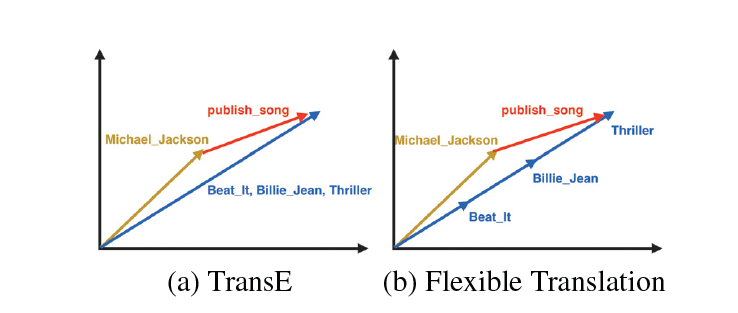得分函数同时衡量了$h+t$和$t$，$t-h$和$h$的方向：
$f(h, r, t)=(h+r)^{T} t+(t-r)^{T} h$

TransA TransA: An Adaptive Approach for Knowledge Graph Embedding(2015)
作者首先提出了两个TransE模型的不足：

loss metric：TransE的loss metric为$\|\mathbf{h}+\mathbf{r}-\mathbf{t}\|_{2}^{2}$，是一种欧氏距离的计算，其对应的图形是一个圆。由于这种简单的loss metric导致不能很好处理复杂的关系（如一对多、多对一等）。论文中给出一个详细案例，从下图可知蓝色部分为正例，红色部分为负例，TransE 模型划分错了七个点。而本文提出了一种基于马氏距离的 TransA 模型，其 PCA 降维图形对应的是一个椭圆，该模型只分错了三个点。identical dimension：TransE等之前的模型等价地对待向量中的每一维度，但是实际上各个维度的重要度是不相同的，有些维度是有作用的，有些维度却是噪音。还是以论文中的栗子，对于关系HasPart来说，左边的TransE模型根据欧氏距离计算得出(room, haspart, goniff)这样错误的三元组。通过对x，y轴进行分解之后发现，关系HasPart会更关注与x轴相近的实体。TransA模型为此引入了对每一维度的加权矩阵。模型
TransA模型对之前的打分函数进行了改进，引入加权矩阵$W_{r}$：$f_{r}(h, t)=(|\mathbf{h}+\mathbf{r}-\mathbf{t}|)^{\top} \mathbf{W}_{\mathbf{r}}(|\mathbf{h}+\mathbf{r}-\mathbf{t}|)$并通过LDL对$W_{r}$进行分解：$\mathbf{W}_{\mathbf{r}}=\mathbf{L}_{\mathbf{r}}^{\top} \mathbf{D}_{\mathbf{r}} \mathbf{L}_{\mathbf{r}}$整理后得到最终的打分函数：$f_{r}=\left(\mathbf{L}_{\mathbf{r}}|\mathbf{h}+\mathbf{r}-\mathbf{t}|\right)^{\top} \mathbf{D}_{\mathbf{r}}\left(\mathbf{L}_{\mathbf{r}}|\mathbf{h}+\mathbf{r}-\mathbf{t}|\right)$训练过程损失函数\begin{aligned} \min & \sum_{(h, r, t) \in \Delta\left(h^{\prime}, r^{\prime}, t^{\prime}\right) \in \Delta^{\prime}}\left[f_{r}(h, t)+\gamma-f_{r^{\prime}}\left(h^{\prime}, t^{\prime}\right)\right]_{+}+\\ & \lambda\left(\sum_{r \in R}\left\|\mathbf{W}_{\mathbf{r}}\right\|_{F}^{2}\right)+C\left(\sum_{e \in E}\|\mathbf{e}\|_{2}^{2}+\sum_{r \in R}\|\mathbf{r}\|_{2}^{2}\right) \\ \text { s.t. } &\left[\mathbf{W}_{\mathbf{r}}\right]_{i j} \geq 0 \end{aligned}

KG2E Learning to Represent Knowledge Graphs with Gaussian Embedding(2015)
作者指出TransE、TransR等之前的研究，在分离正三元组及其对应的负三元组时，不同的实体和关系往往共享相同的边界，而知识库中实体和关系的**（非）确定性**被完全忽略。但实际上，不同的实体和关系常常包含不同的确定性。在这里，一个实体/关系的（非）确定性表示在将一个三元组作为上下文评分时表示其语义的可信度。举个栗子，在推断一个人时，配偶关系的确定性明显大于国籍。在预测Hillary Clinton时，当我们知道她丈夫（配偶）是Bill Clinton时，我们可能更有信心知道她是谁，而不是知道她出生在（国籍）美国。
为此，本文使用Gaussian Distribution 来表示实体和关系，使用协方差来表示实体和关系的不确定度。相同颜色的圆圈代表同意三元组，圆圈大小则表示不同三元组的不确定度。相对于之前的point-based model，本文的KG2E是一种density-based model。在建模过程中强调了(un)certainty的概念，融入关系和实体语义本身的不确定性并使用高斯分布协方差表示实体关系的不确定度，使用高斯分布的均值表示实体或关系在语义空间中的中心值。

TransG TransG : A Generative Model for Knowledge Graph Embedding(2016)
本文主要是为了解决多关系语义（multiple relation semantics），即某一种关系可能会存在多个类别的语义，比如对于(Atlantics, HasPart, NewYorkBay)和(Table, HasPart, Leg)两个三元组都属于关系HasPart，但是他们在语义上并不相同，一个是位置的语义，一个是部件的语义。其实这个问题在之前介绍的CTransR中也提出过解决方案，但是作者似乎认为CTransR预处理聚类的操作不是很elegant。为此，作者们提出了一种基于贝叶斯非参的无限混和嵌入模型：认为关系向量由若干子成分向量合成，模型会根据实体对自动选择一个关系向量，而多少个这样的关系向量还可以由模型自动选择。 整体过程如下：一张总结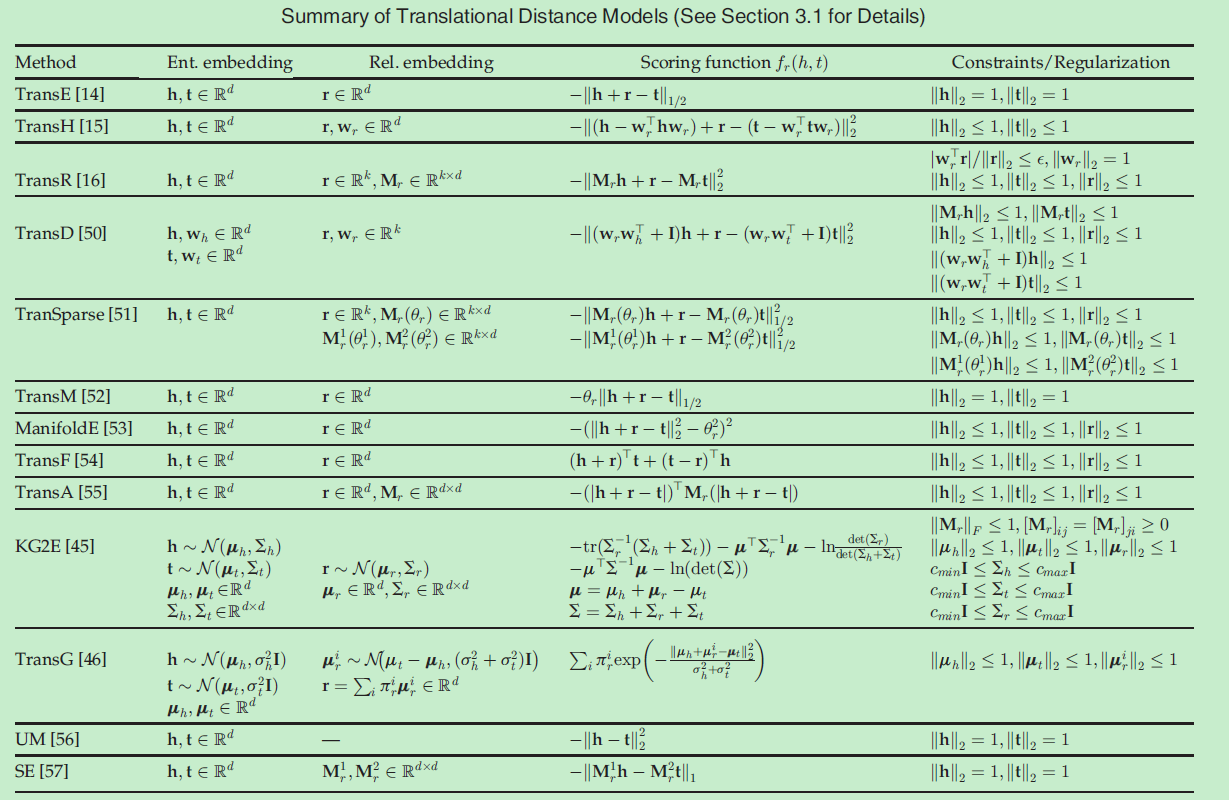以上
2019.10.08


展开全文Trans系列
• ## 稀疏表示学习

万次阅读 2017-04-25 21:22:42
1.提出问题：什么是稀疏表示 假设我们用一个M*N的矩阵表示数据集X，每一行代表一个样本，每一列代表样本的一个属性，一般而言，该矩阵是稠密的，即大多数元素不为0。 稀疏表示的含义是，寻找一个系数矩阵A（K*N...


1.提出问题：什么是稀疏表示
假设我们用一个M*N的矩阵表示数据集X，每一行代表一个样本，每一列代表样本的一个属性，一般而言，该矩阵是稠密的，即大多数元素不为0。
稀疏表示的含义是，寻找一个系数矩阵A（K*N）以及一个字典矩阵B（M*K），使得B*A尽可能的还原X，且A尽可能的稀疏。A便是X的稀疏表示。

书上原文为（将一个大矩阵变成两个小矩阵，而达到压缩）

“为普通稠密表达的样本找到合适的字典，将样本转化为合适的稀疏表达形式，从而使学习任务得以简化，模型复杂度得以降低，通常称为‘字典学习’（dictionary learning），亦称‘稀疏编码’（sparse coding）”块内容

表达为优化问题的话，字典学习的最简单形式为：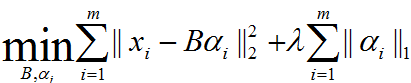其中xi为第i个样本，B为字典矩阵，aphai为xi的稀疏表示，lambda为大于0参数。
上式中第一个累加项说明了字典学习的第一个目标是字典矩阵与稀疏表示的线性组合尽可能的还原样本；第二个累加项说明了alphai应该尽可能的稀疏。之所以用L1范式是因为L1范式正则化更容易获得稀疏解。具体原因参看该书11.4章或移步机器学习中的范数规则化之（一）L0、L1与L2范数。字典学习便是学习出满足上述最优化问题的字典B以及样本的稀疏表示A（A{alpha1，alpha2，…，alphai}）。L1正则化常用于稀疏，可以获得稀疏解。如下图表示，L1正则化交点在轴上，所得的解一般只是在某个轴上有实数，另外的轴为0，从而最终得到稀疏解。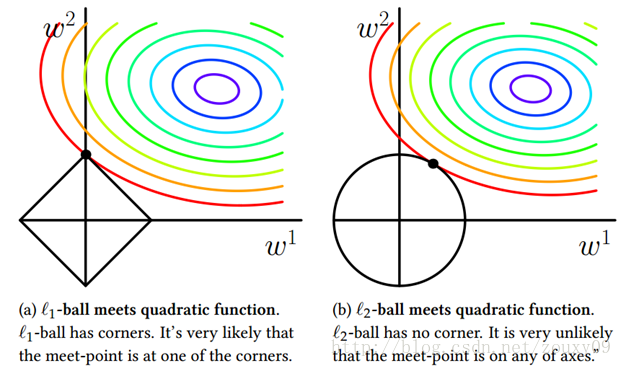2.字典学习求解 （学习字典、稀疏表示）
求解上述最优化问题的总体策略是，对字典B以及样本稀疏表示alphai交替迭代优化。即先初始化字典B，
1.固定字典B对alphai进行优化。2.固定A对字典B进行优化。重复上述两步，求得最终B以及X的稀疏表示A。
参考：
http://blog.csdn.net/sam92/article/details/50731607
http://blog.csdn.net/zouxy09/article/details/24971995/


展开全文• ## 语言表示学习

千次阅读 2016-12-21 21:02:27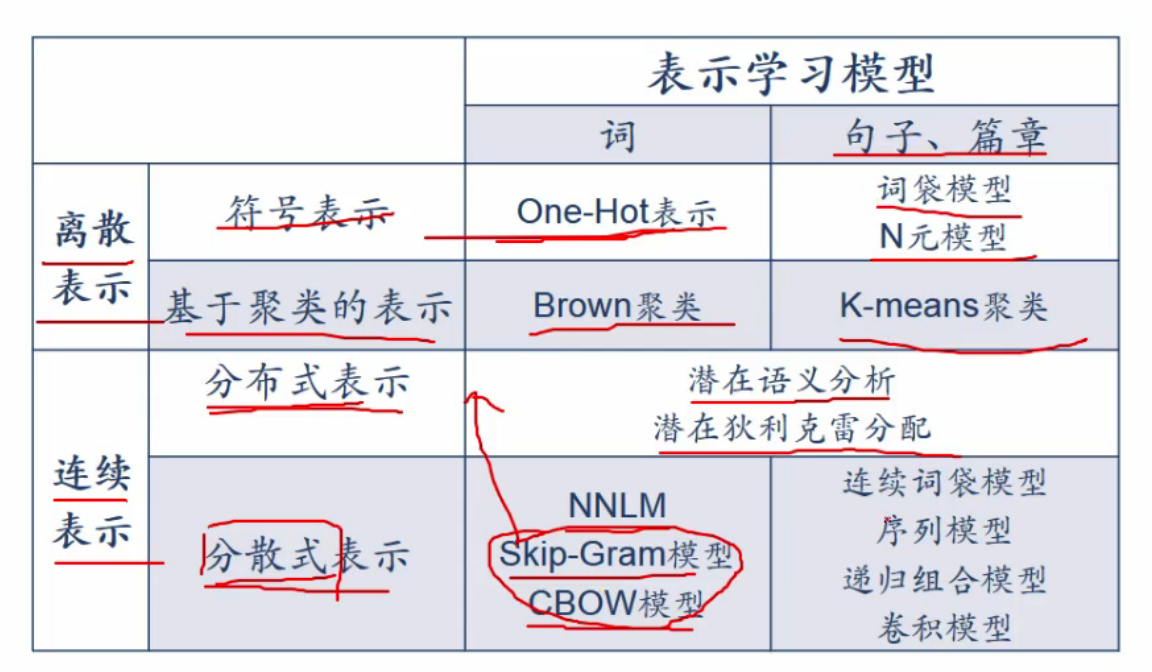展开全文• ## 网络表示学习

千次阅读 2018-06-20 17:02:31
Network Representation Learning, 网络表示学习. 类似于word2vec, 目标是用低维、稠密、实值的向量表示网络中的节点, 方便下游计算. 基本思想 仍旧类比于word2vec. 使用DFS/BFS的搜索思想对图进行遍历, 得到...
• 深度学习是表示学习的经典代表： 深度学习的过程： 深度学习与传统机器学习差别： 深度学习代表算法： 先来看机器学习： 机器学习是利用经验experience来改善 计算机系统自身的性能，通过经验获取知识knowledge...
• ## representation learning 表示学习

万次阅读 多人点赞 2017-12-17 15:47:53
• ## 字典学习/稀疏表示学习笔记

万次阅读 多人点赞 2016-02-24 16:10:58
首先向大家安利一下南大周志华老师写的《机器学习》这本书，作为一个对此一窍不通的人看了都觉得很有意思，受益匪浅。语言平实却又干货十足，比某些故弄玄虚泛泛而谈的其它国内教材高到不知哪里去了。 最近看的...语言
• ## 网络表示学习总结

万次阅读 2017-07-03 10:47:27
最近看了paperweekly的两次关于网络表示学习的直播，涂存超博士与杨成博士讲解了网络表示学习的相关知识。本文将网络表示学习中的一些基本知识，结合自己的一些粗浅的理解，整理记录下来。
• ## 知识图谱表示学习

千次阅读 2018-06-26 10:49:03
知识图谱是一种精细化的异构网络, 所以对其节点与边的表示学习也是一个热门的问题. 这里的学习依旧是得到它们的低维稠密向量. 参考 论文transr
• ## 网络表示学习笔记

千次阅读 2018-04-12 19:57:09
网络表示学习（Representation Learning on Network），一般说的就是向量化（Embedding）技术，简单来说，就是将网络中的结构（节点、边或者子图），通过一系列过程，变成一个多维向量，通过这样一层转化，能够将...
• 通过网络的权值来捕捉外界输入模式的特征，并且通过网络连接方式来组合这些特征从而提取出更加高层特征，采用这种方法逐级从大量的输入数据中学习到对于输入模式有效的特征表示，然后再把学习到的特征用于分类、回归...
• ## 表示学习(Representation Learning)

万次阅读 多人点赞 2019-05-10 14:07:39
2013年，Bengio等人发表了关于表示学习的综述。最近拜读了一下，要读懂这篇论文还有很多文献需要阅读。组会上正好报了这篇，所以在此做一个总结。 二、表示学习发展由来 当我们学习一个复杂概念时，总想有一条...
• Paper: 刘知远 2016 知识表示学习研究进展 计算机研究与发展 1. 表示学习旨在将研究对象的语义信息表示为稠密低维表示向量；而知识表示学习则面向知识库中的实体和关系进行表示学习；该技术可以在低维空间中高效...知识图谱
• 本章的表示学习主要就是通过无监督训练学习特征的意思。 这种没有具体的算法，就是介绍表示学习的应用和大概的分支，至于如何进行表示学习，没有详细介绍。感觉可以直接跳过。。 贪心逐层无监督预训练 贪心算法在...
• 本文转载自公众号：人工智能图灵。刘知远，清华大学计算机系副教授、博士生导师。主要研究方向为表示学习、知识图谱和社会计算。2011年获得清华大学博士学位，已在ACL、IJC...
• 在机器学习领域，表征学习（或特征学习）是一种将原始数据转换成为能够被机器学习有效开发的一种技术的集合。在特征学习算法出现之前，机器学习研究人员需要利用手动特征工程（manual feature learning）等技术从...
• 网络表示学习（network representation learning，NRL）,也被称为图嵌入方法（graph embedding method，GEM）是这两年兴起的工作，目前很热，许多直接研究网络表示学习的工作和同时优化网络表示+下游任务的工作正在...
• ## [ 解耦表示学习 ]

千次阅读 2019-07-11 15:27:06
上图转载自：https://blog.csdn.net/dQCFKyQDXYm3F8rB0/article/details/89899601
• 目前大多数图嵌入方法在训练过程中需要图中所有节点参与，属于直推学习（transductive），无法直接泛化到之前未见的节点。本文提出一种适用于大规模网络的归纳式（inductive）模型-GraphSAGE，能够为新增节点快速...
• 最新语言表示学习方法XLNet，在20项任务上超越BERT 摘要：凭借对双向上下文进行建模的能力，与基于自回归语言建模的预训练方法相比，基于BERT的基于自动编码的预训练实现了更好的性能。然而，依赖于对输入使用掩码...
• dataset #relation #entity # triple(train/valild/test) WN11 11 38696 112581 &amp;nbsp; &amp;nbsp; 2609 &amp;nbsp;&amp;nbsp; 10544 WN18 18 40943 141442 &amp;...13
• Zachary’s karate club 一个大学空手道俱乐部的社交关系图, 很多论文中都喜欢用它做例子. 这个图比较简单, 有34个节点, 78条边. youtube 2 数据集介绍见....node有两种, personpersonperson 与 groupgroupgroup...
• ## 表示学习2-Deep Walk

千次阅读 2017-09-20 14:34:52
• DeepNLP的表示学习·词嵌入来龙去脉·深度学习（Deep Learning）·自然语言处理（NLP）·表示（Representation） 词向量、word embedding、分布式表示、word2vec、glove 术语解释！ 自然语言处理表示学习 词向量、...
• Structural Deep Network Embedding 作者：Daixin Wang, Peng Cui, Wenwu Zhu 刊物：KDD ’16, August 13-17, 2016, San Francisco, CA, USA ...几乎所有现有的网络表示方法采用的都是浅层模型。然...
• 【新智元导读】今天，DeepMind的一篇新论文引发学术圈热烈反响——基于最强图像生成器BigGAN，打造了BigBiGAN，在无监督表示学习和图像生成方面均实现了最先进的性能！Ian Goodfellow也称赞“太酷了！” 生成对抗......